# A positive charge is moving with velocity v through a region of space where a uniform...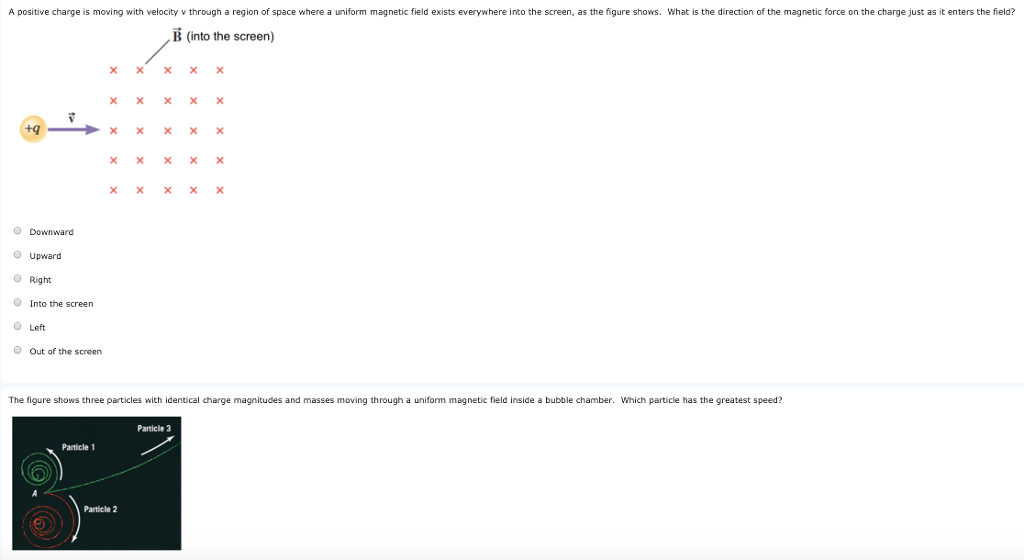A positive charge is moving with velocity v through a region of space where a uniform magnetic field exists everywhere into the screen, as the figure shows. What is the direction of the magnetic force on the charge just as it enters the field? B (into the screen) ㄨㄨㄨㄨㄨ ㄨㄨㄨㄨㄨ Downward Upward O Right O Into the screen Left O Out of the screen The figure shows three particles with identical charge magnitudes and masses moving through a uniform magnetic field inside a bubble chamber. Which particle has the greatest speed? Particle 3 Particle 1 Particle 2

The right hand rule states that, to find the direction of the magnetic force on a positive moving charge, the thumb of the right hand point in the direction of v, the fingers in the direction of B, and the force (F) is directed perpendicular to the right hand palm.

#### Earn Coin

Coins can be redeemed for fabulous gifts.

Similar Homework Help Questions
• ### A positive charge is moving with velocity v through a region of space where a uniform...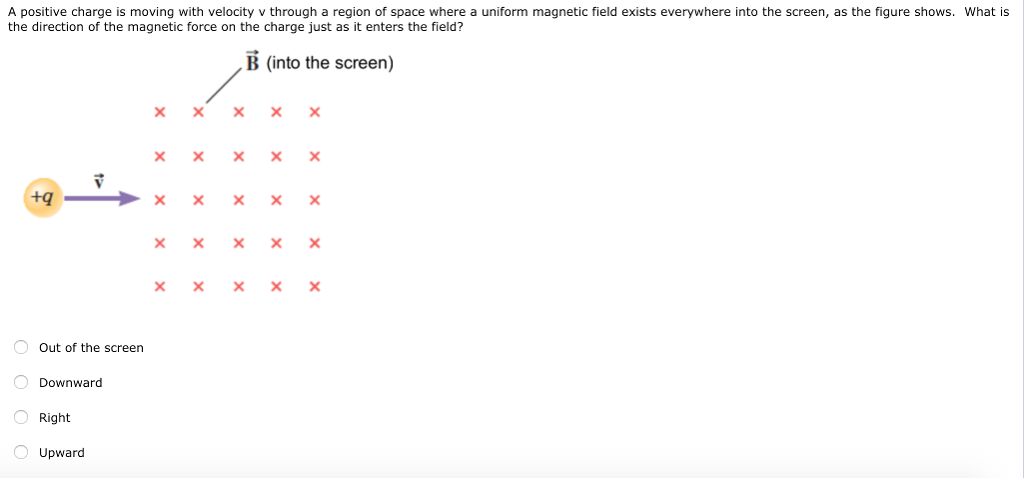A positive charge is moving with velocity v through a region of space where a uniform magnetic field exists everywhere into the screen, as the figure shows. What is the direction of the magnetic force on the charge just as it enters the field? B (into the screen) ㄨㄨㄨㄨㄨ ㄨㄨㄨㄨㄨ ㄨㄨㄨㄨㄨ Out of the screen Downward Right Upward

• ### The figure shows three particles with identical charge magnitudes and masses moving through a uniform magnetic...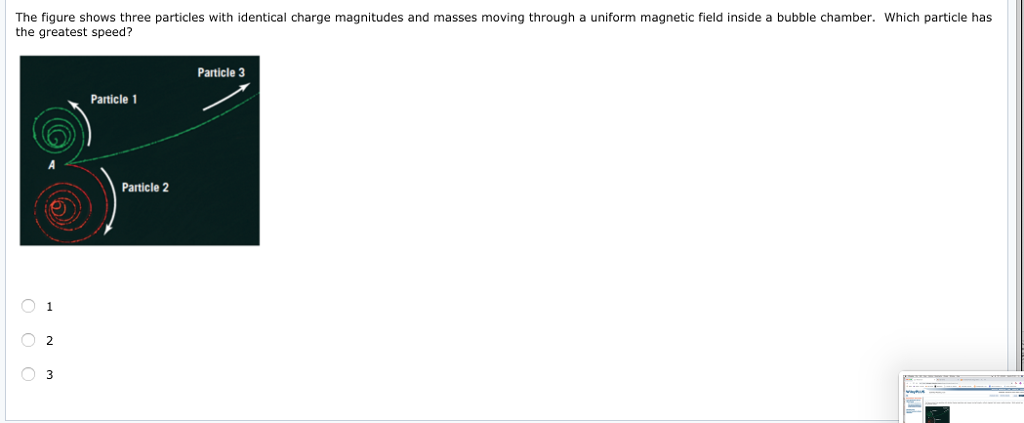The figure shows three particles with identical charge magnitudes and masses moving through a uniform magnetic field inside a bubble chamber. Which particle has the greatest speed? Particle 3 Particle 1 Particle 2 2

• ### Particle roundabout. The figure shows 11 paths through a region of uniform magnetic field. One path...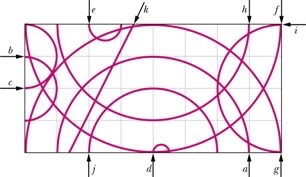Particle roundabout. The figure shows 11 paths through a region of uniform magnetic field. One path is a straight line; the rest are half-circles. The table gives the masses, charges, and speeds of 11 particles that take these paths through the field in the directions shown. Which path in the figure corresponds to which particle in the table?

• ### A positively charged particle is moving with a constant velocity directed to the right through a...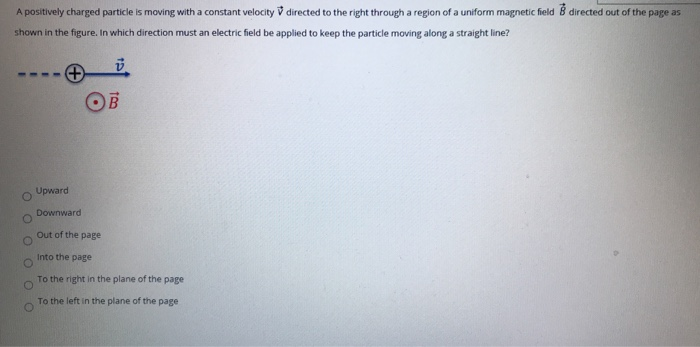A positively charged particle is moving with a constant velocity directed to the right through a region of a uniform magnetic field 8 directed out of the page as shown in the figure. In which direction must an electric field be applied to keep the particle moving along a straight line? OB Upward Downward Out of the page Into the page To the right in the plane of the page To the left in the plane of the page

• ### A proton (charge = +e) instantaneously is moving north in a region where there is a...

A proton (charge = +e) instantaneously is moving north in a region where there is a uniform magnetic field pointing downward. At this instant, in what direction will the proton be deflected? to the east no direction (zero force) upward to the west downward

• ### An electron traveling left to right enters a region of space where a uniform magnetic field...

An electron traveling left to right enters a region of space where a uniform magnetic field exists (see figure). Once in the field, the electron follows a circular path where r = 1.0E-6 m. If the magnitude of the magnetic field is 5.5 T, what is the speed of the electron and the direction of the magnetic field? 530 m/s, into the screen 530 m/s, out of the screen 9.7E5 m/s, into the screen 9.7E5 m/s, out of the screen...

• ### A proton is released from rest at a location in space where the magnetic field points...A proton is released from rest at a location in space where the magnetic field points upward toward the top of the page. Which of the following is true? The proton experiences no magnetic force and remains at rest. The proton experiences a magnetic force upward. The proton experiences a magnetic force downward. The proton experiences a magnetic force to the right. The figure shows three identical charged particles moving with the same speed but at different directions in a...

• ### A particle of charge and mass moves in a region of space where there is a uniform magnetic field.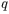A particle of charge and mass moves in a region of space where there is a uniform magnetic field (i.e., a magnetic field of magnitude in the +z direction).In thisproblem,neglect any forces on the particle other than the magneticforce.At a given moment the particle is moving inthe +x direction (and the magnetic field is always in the+z direction). If is positive, what is the direction of the force on the particle dueto the magnetic field?+x-x+y-y+z-z

• ### There is a uniform magnetic field which points to the right. A+2 C charge is moving...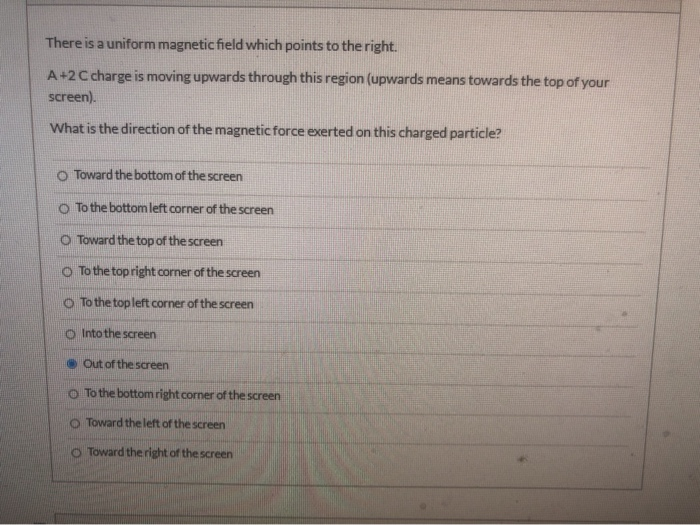There is a uniform magnetic field which points to the right. A+2 C charge is moving upwards through this region (upwards means towards the top of your screen). What is the direction of the magnetic force exerted on this charged particle? O Toward the bottom of the screen To the bottom left corner of the screen Toward the top of the screen To the top right corner of the screen To the topleft corner of the screen Into the screen...

• ### three particles having the same mass and same horizontal velocity enter a region of constant magnetic...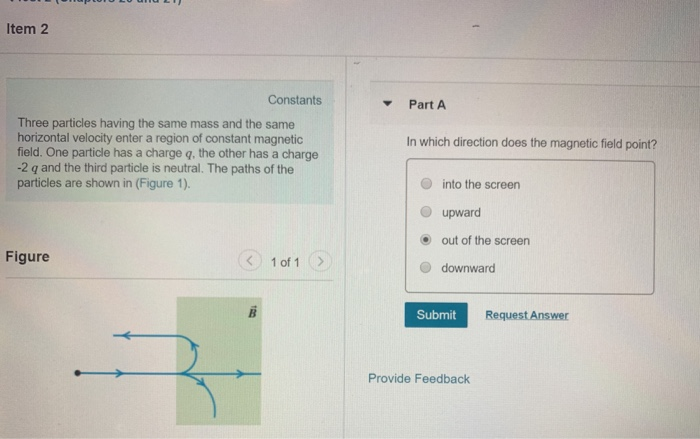three particles having the same mass and same horizontal velocity enter a region of constant magnetic field. ( i know the answer for if you had q and -4q is out of the screen) Item 2 Constants PartA Three particles having the same mass and the same horizontal velocity enter a region of constant magnetic field. One particle has a charge q, the other has a charge -2 q and the third particle is neutral. The paths of the particles...